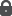Predictor-corrector algorithm for solving P_* (k)-matrix LCP from arbitrary positive starting points
Potra, Florian A.
Rongqin, Sheng

 Data: 1997 Resum: A new predictor-corrector algorithm is proposed for solving P_*(k)-matrix linear complementarity problems. If the problem is solvable, then the algorithm converges from an arbitrary positive starting point (x^0, s^0). The computational complexity of the algorithm depends on the quality of the starting point. If the starting point is feasible or close to being feasible, it has O((1 + k) V~n/(rho)_0L)-iteration complexity, where (rho)_0 is the ratio of the smallest and average coordinate of X^0s^0. With appropriate initialization, a modified version of the algorithm terminates in O((1 + k)^2 (n/(rho)_0)L) steps either by finding a solution or by determining that the problem has no solution in a predetermined, arbitrarily large, region. The algorithm is quadratically convergent for problems having a strictly complementary solution. We also propose an extension of a recent algorithm of Mizuno to P_* (k)-matrix linear complementarity problems such that it can start from arbitrary positive points and has superlinear convergence without a strictly complementary condition. . Drets: Tots els drets reservats.Llengua: Anglès Document: Article ; recerca ; Versió publicada Matèria: Linear complementarity problems ; P_*-matrices ; Interior-point algorithm ; Superlinear convergence Publicat a: Mathematical Programming, vol. 76 n. 1 (1997) p. 223-244, ISSN 0025-5610

 22 p, 782.7 KBAccés restringit a la UAB

El registre apareix a les col·leccions:
Articles > Articles de recerca
Articles > Articles publicats

Registre creat el 2006-03-13, darrera modificació el 2022-09-10

 #bookmark_sciencewise, #bookmark { float: left; } #bookmark_sciencewise li { padding: 2px; width: 25px} #bookmark_sciencewise ul, #bookmark ul { list-style-image: none; } @import "https://ddd.uab.cat/css/jquery.bookmark.css";Afegeix-lo al cistell personal Anomena i desa Citation, BibTeX, MARC, MARCXML, DC, EDM OpenAire4 #bookmark_sciencewise, #bookmark { float: left; } #bookmark_sciencewise li { padding: 2px; width: 25px} #bookmark_sciencewise ul, #bookmark ul { list-style-image: none; } @import "https://ddd.uab.cat/css/jquery.bookmark.css";